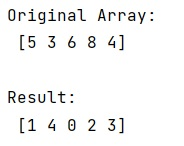# How to get the indices of the sorted array using NumPy?

Learn, how to get the indices of the sorted array using NumPy in Python?
Submitted by Pranit Sharma, on February 26, 2023

NumPy is an abbreviated form of Numerical Python. It is used for different types of scientific operations in python. Numpy is a vast library in python which is used for almost every kind of scientific or mathematical operation. It is itself an array which is a collection of various methods and functions for processing the arrays.

## Getting the indices of the sorted array using NumPy

Suppose that we are given a numpy array of some numerical values (int or float). We need to get the indices of the sorted array which means we need the elements sorted but their indices according to the original order.

For example, we have an array [1,3,5,2] where the index of 1 is 0, 3 is 1, 5 is 2, and 2 is 3, we need the indices in such an order that the elements are sorted but their indices are original, in this case, it would be [0,3,1,2].

For this purpose, we can call argsort() as a method on ndarray object, it does exactly what we want. It returns the original indices of the sorted array.

Let us understand with the help of an example,

## Python code to get the indices of the sorted array using NumPy

```# Import numpy
import numpy as np

# Creating a numpy array
arr = np.array([5,3,6,8,4])

# Display original array
print("Original Array:\n",arr,"\n")

# Getting the original indices of
# sorted array
res = arr.argsort()

# Display result
print("Result:\n",res)
```

Output:Languages: » C » C++ » C++ STL » Java » Data Structure » C#.Net » Android » Kotlin » SQL
Web Technologies: » PHP » Python » JavaScript » CSS » Ajax » Node.js » Web programming/HTML
Solved programs: » C » C++ » DS » Java » C#
Aptitude que. & ans.: » C » C++ » Java » DBMS
Interview que. & ans.: » C » Embedded C » Java » SEO » HR
CS Subjects: » CS Basics » O.S. » Networks » DBMS » Embedded Systems » Cloud Computing
» Machine learning » CS Organizations » Linux » DOS
More: » Articles » Puzzles » News/Updates